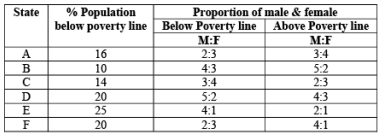Following table shows the percentage population of six states below poverty line and proportion of male and female.Q. If the population of males below poverty line in state C is 6000 & state E 1000 then what is the ratio of the total population of state C& E is
• a)
2: 1
• b)
3: 5
• c)
11: 5
• d)
NOTA

### Related TestAditya Sharma Jul 26, 2019
APioneer Academy Feb 18, 2022The ratio of Males and Females below poverty line in C is 3 : 4. Since there are 6000 males below poverty line in C, so there will be 8000 females below poverty line. Hence total people below poverty line in C = 6000 + 8000 = 14000. Now this is 14% of total population.
Hence 14% of total population = 14000
∴ Total pop = 100000
The no. of males below poverty line in E is 10000 and the ratio is 4 : 1. Hence there are 2500 females in E below poverty line. So total people below poverty line is 12500 which is 25% of total population.
Hence total population of state E = 50000
Hence the reqd. ratio is 100000 : 50000 or 2 : 1

The ratio of Males and Females below poverty line in C is 3 : 4. Since there are 6000 males below poverty line in C, so there will be 8000 females below poverty line. Hence total people below poverty line in C = 6000 + 8000 = 14000. Now this is 14% of total population.Hence 14% of total population = 14000∴ Total pop = 100000The no. of males below poverty line in E is 10000 and the ratio is 4 : 1. Hence there are 2500 females in E below poverty line. So total people below poverty line is 12500 which is 25% of total population. Hence total population of state E = 50000Hence the reqd. ratio is 100000 : 50000 or 2 : 1
Do you know? How Toppers prepare for CAT Exam
With help of the best CAT teachers & toppers, We have prepared a guide for student who are preparing for CAT : 15 Steps to clear CAT Exam